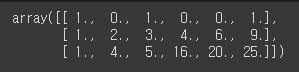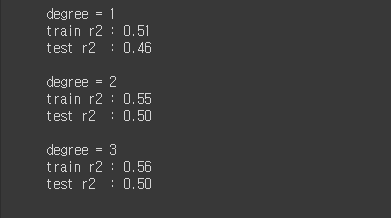# Multiple Linear Regression

tjddyd1592·2021년 4월 6일
0

목록 보기
4/12

## 학습내용

### 무작위 샘플링

train = df.sample(frac = 0.75, random_state=1)
test = df.drop(train.index)

### target, feature / train, test 데이터셋 생성

feature = []
target = []

X_train = train[feature]
X_test = test[feature]
y_train = train[target]
y_test = test[target]

from sklearn.model_selection import train_test_split

rng = np.random.RandomState(1)
data = np.dot(rng.rand(2, 2), rng.randn(2, 30)).T
X = pd.DataFrame([i for i in data])
y = pd.DataFrame([i for i in data])

#함수를 이용하는 방법
X_train, X_test, y_train, y_test = train_test_split(X,y, random_state = 1)

### 학습 및 검정

from sklearn.metrics import mean_absolute_error

model = LinearRegression

model.fit(X_train, y_train)
y_pred_test = model.predict(X_test)
y_pred_tarin = model.predict(X_train)

mean_absolute_error(y_test, y_pred_test) #테스트 에러
mean_absolute_error(y_train, y_pred_train) #training 에러

model.score(X_train, y_train) #model의 속성이용 r2
r2_score(y_test, y_pred) #r2_score 이용 r2

### 다항회귀모델의 차수 조정

from sklearn.preprocessing import PolynomialFeatures

X1 = np.arange(6).reshape(3,2)

poly = PolynomialFeatures(2)
X_poly = poly.fit_transform(X1)
## X_poly: [1, a, b, a^2, ab, b^2]
X_poly### PolynomialRegression

from sklearn.linear_model import LinearRegression
from sklearn.metrics import r2_score
from sklearn.pipeline import make_pipeline
from sklearn.preprocessing import PolynomialFeatures
import matplotlib.pyplot as plt

#Polynomial regression

from sklearn.pipeline import make_pipeline
from sklearn.preprocessing import PolynomialFeatures

def PolynomialRegression(dgree = 2):
return make_pipeline(PolynomialFeatures(degree), LinearRegression())
#연속된 변환을 순차적으로 처리할 수 있는 기능을 제공하는 유용한 래퍼(Wrapper) 도구
#for 문으로 처리되는 과정 확인 가능

degrees = [1,2,3]

for degree in degrees:
model2 = PolynomialRegression(degree)
print('degree = {}'.format(degree))

model2.fit(X_train,y_train)

train_r2 = model2.score(X_train, y_train) #model.score로 r2값 바로 계산 가능
test_r2 = model2.score(X_test, y_test)

print('train r2 : {:.2f}'.format(train_r2))
print('test r2  : {:.2f}'.format(test_r2))
print()### 그래프

import matplotlib.pyplot as plt
import seaborn as sns
import plotly.express as px
import plotly.graph_objs as go

#3d
px.scatter_3d(
data,
x = ,
y = ,
z = ,
title = ''

#### 추가내용

def print_kwargs(**kwargs):
print(kwargs)
# 입력을 딕셔너리 형태로 돌려줌